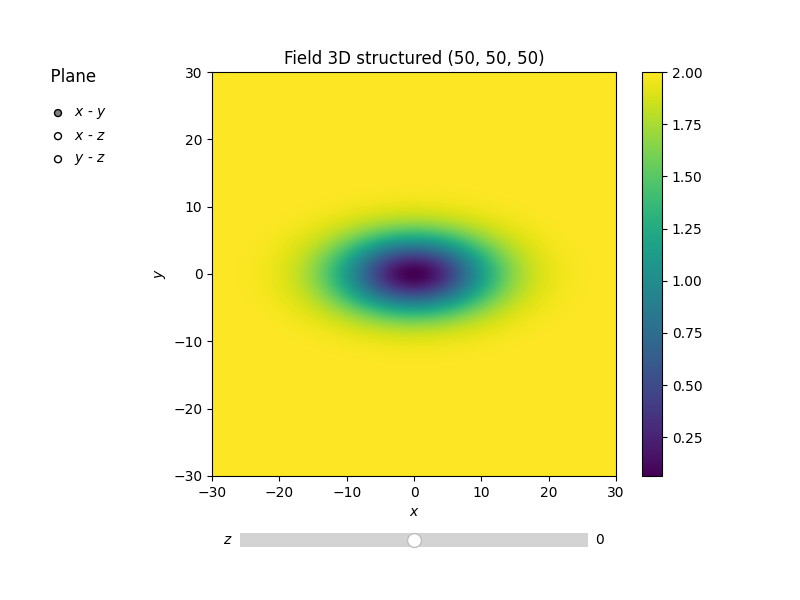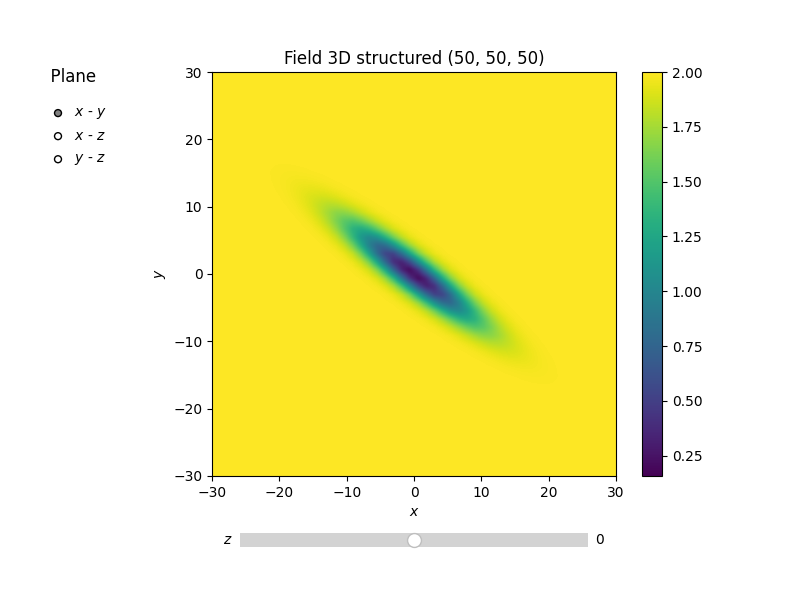# Anisotropy and Rotation¶

The internally used (semi-) variogram represents the isotropic case for the model. Nevertheless, you can provide anisotropy ratios by:

```import gstools as gs

model = gs.Gaussian(dim=3, var=2.0, len_scale=10, anis=0.5)
print(model.anis)
print(model.len_scale_vec)
```

Out:

```[1.  0.5]
[10. 10.  5.]
```

As you can see, we defined just one anisotropy-ratio and the second transversal direction was filled up with `1.`. You can get the length-scales in each direction by the attribute `CovModel.len_scale_vec`. For full control you can set a list of anistropy ratios: `anis=[0.5, 0.4]`.

Alternatively you can provide a list of length-scales:

```model = gs.Gaussian(dim=3, var=2.0, len_scale=[10, 5, 4])
model.plot("vario_spatial")
print("Anisotropy representations:")
print("Anis. ratios:", model.anis)
print("Main length scale", model.len_scale)
print("All length scales", model.len_scale_vec)
```Out:

```Anisotropy representations:
Anis. ratios: [0.5 0.4]
Main length scale 10
All length scales [10.  5.  4.]
```

## Rotation Angles¶

The main directions of the field don’t have to coincide with the spatial directions,and. Therefore you can provide rotation angles for the model:

```model = gs.Gaussian(dim=3, var=2.0, len_scale=[10, 2], angles=2.5)
model.plot("vario_spatial")
print("Rotation angles", model.angles)
```Out:

```Rotation angles [2.5 0.  0. ]
```

Again, the angles were filled up with `0.` to match the dimension and you could also provide a list of angles. The number of angles depends on the given dimension:

• in 1D: no rotation performable
• in 2D: given as rotation around z-axis
• in 3D: given by yaw, pitch, and roll (known as Tait–Bryan angles)

Total running time of the script: ( 0 minutes 1.312 seconds)

Gallery generated by Sphinx-Gallery